Disclosure: This post may contain affiliate links, meaning when you click the links and make a purchase, we receive a commission.

# How to calculate salary increase percentage in Excel [Free Template]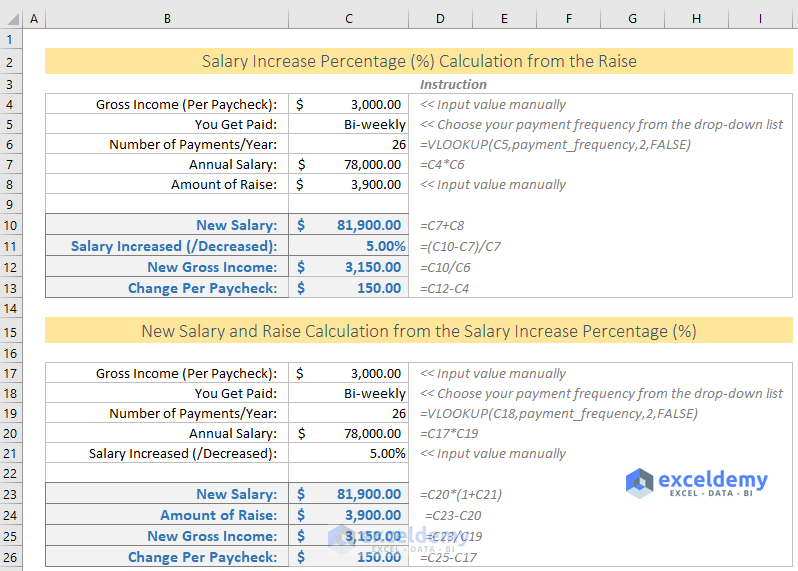In this short tutorial, I will show you how to calculate salary increase percentage (%) in Excel from your recent raise.

Also, you will learn how to calculate the amount of raise from the salary increase percentage (%).

In every calculation, you will be able to see the amount of difference made in your per paycheck.

Calculate-salary-increase-percentage-in-Excel.xlsx

## Salary Increase Percentage (%) Calculation from the Raise

From your Paycheck Stub, you will take the Gross Salary. Don’t deduct anything from the Gross Salary like Medical Tax, Social Security Tax, Fed Tax or anything else. Normally Gross Salary and Deductions are shown in the different column. So, it will be easy for you to find out the Gross Wages from the paycheck stub.

A sample Paycheck stub.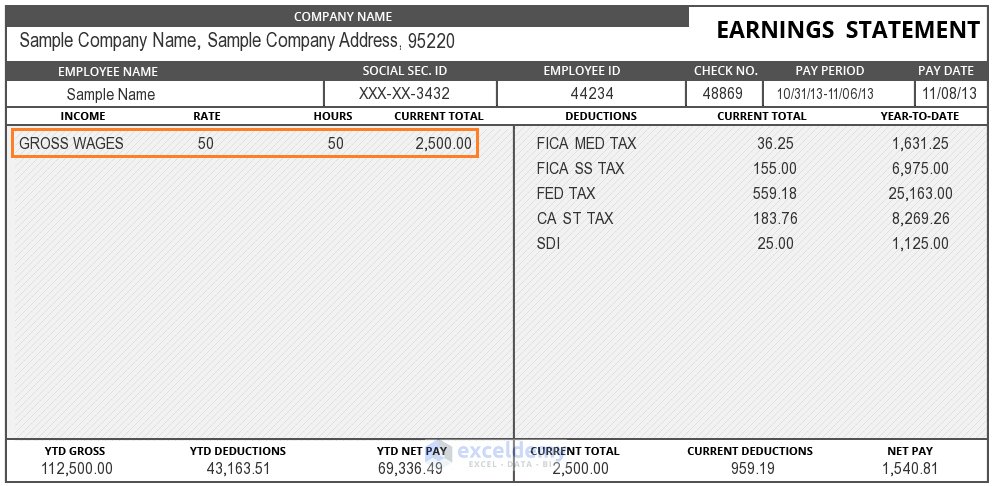In the following image, you’re seeing the whole process I have used to calculate the Salary Increase Percentage from the Salary Raise.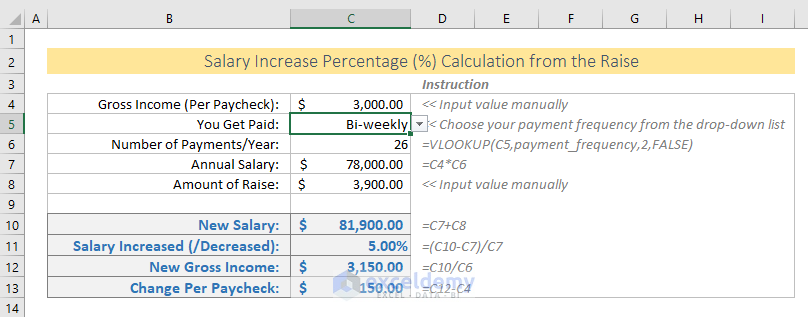Input / Output values in the Excel Template:

• Gross Income (Per Paycheck): Input your Gross Income value in the cell C4.
• You Get Paid: This is a drop-down list. Input your payment frequency. Though I have input a lot of values in the list, normally employees are paid Weekly, Bi-weekly and Monthly.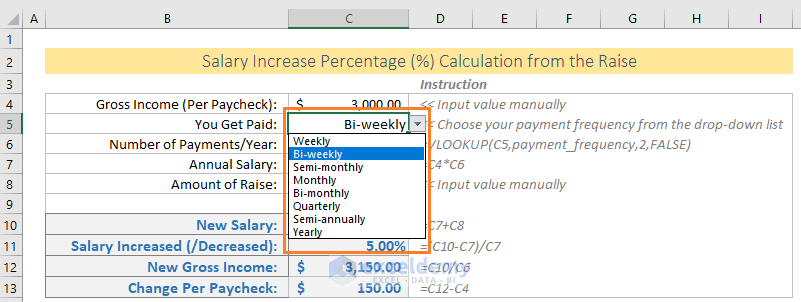• Number of Payments/Year: This is the value you will get from a VLOOKUP table. In the Payments worksheet (a hidden worksheet), you will get a range named as payment_frequency. We have applied VLOOKUP formula to get the Payment Frequency in a year.

=VLOOKUP(C5,payment_frequency,2,FALSE)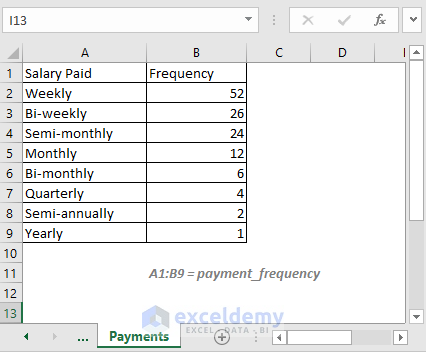• Annual Salary: This is also an output. We got it multiplying the Gross Income (Per Paycheck) by the Number of Payments per Year: =C4*C6
• Amount of Raise: It will be input by you. Input the raise you have been provided by your company in the cell C8.
• New Salary: Your new salary will be the sum of your old Annual Salary and Raise: =C7 + C8
• Salary Increased (/Decreased): It will be calculated by this formula:

=(C10-C7)/C7

= (New Annual Salary – Old Annual Salary)/Old Annual Salary

The cell is formatted with the Percentage format.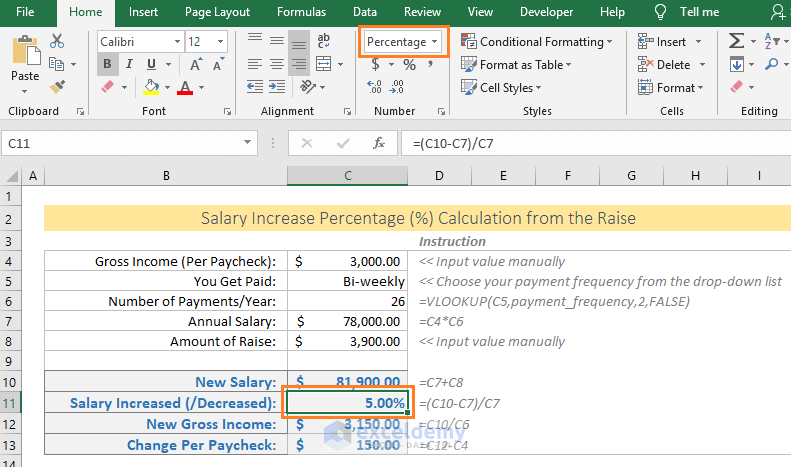• New Gross Income: To get the New Gross Income (per paycheck), you have to divide your new annual income by the total number of payments per year: =C10/C6
• Change Per Paycheck: Just subtract your new Per Paycheck from the old Per Paycheck: =C12-C4

## New Salary and Raise Calculation from the Salary Increase Percentage (%)

In this case, your Salary Increase Percentage will be given, we shall calculate your new Gross Income and Raise.

Check out the following image. You see: this time Salary Increase Percentage is given instead of the Raise amount.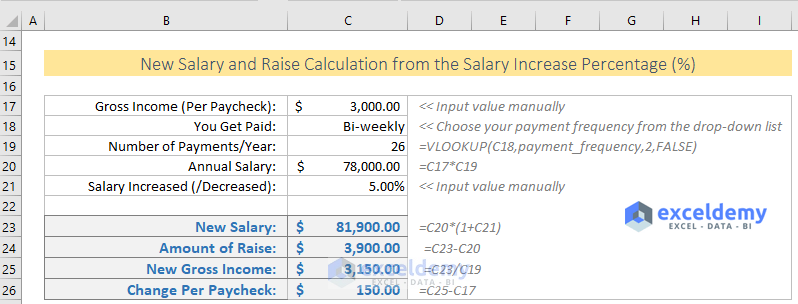Input / Output values in the Excel Template:

• Gross Income (Per Paycheck): Input your Gross Income.
• You Get Paid: Select your payment frequency from the drop-down list.
• Number of Payments/Year: Excel VLOOKUP formula is used to get this value. See the above explanation.
• Annual Salary: Annual Salary is calculated multiplying the Gross Income by the Total Number of Payments per Year.
• Salary Increased (/Decreased): Previously, in this place, we have used the Amount of Raise This time, we are using the percentage increase. You will input this value into the template.
• New Salary: New Salary will be calculated using this formula:

= Old Salary x (1 + Percentage Increase)

= C20*(1+C21)

• Amount of Raise: It is the subtraction of New Annual Salary and Old Annual Salary: =C23-C20
• New Gross Income: Division of the New Annual Salary and the Total Number of Payments per Year: =C23/C19
• Change Per Paycheck: Difference of the New Per Paycheck and the Old Per Paycheck: =C25-C17

## Conclusion

This is how to calculate the salary increase percentage (%) in Excel from Gross Pay and Raise. I have also shown how to calculate the raise from the percentage increase. Hope this article and the Excel template help you.